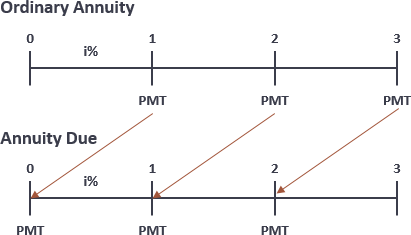# Future Value of Annuity Calculator

The time value of money is the greater benefit of receiving money now rather than an identical sum later. Because of the time value of money, money received or paid out today is worth more than the same amount of money will be in the future. The time value of money explains why interest is paid or earned: interest, whether it is on a bank deposit or debt, compensates the depositor or lender for the time value of money.

## FV vs FVA

• Future Value (FV) refers to the value of a sum of money deposited now, and the final value of the asset worth at a certain point in the future.
• Future Value of Annuity (FVA) refers to the value of an amount of money deposited every certain period from now on, and the final value of the asset worth at a certain point in the future.

## Ordinary Annuities vs Annuities Due

An annuity is a series of payments made or received over a pre-determined period of time.  It can be classified into two basic types: ordinary annuities and annuities due.Ordinary annuities: With an ordinary annuity, payments are made at the end of a covered term. Ordinary annuity payments are usually made monthly, quarterly, semiannually, or annually. For example, bonds generally pay interest at the end of every six months.

Annuities due: With an annuity due, payments are made at the beginning of a covered term rather than at the end. A rent or lease agreement, for instance, is a common example of an annuity due.

## FVA Formula

• `FVA Ordinary = P * [(1 + i)n - 1] / i`
• `FVA Due = P * [(1 + i)n - 1] * (1 + i) / i`

## FVA Example

Suppose an investment of \$15,000 annually deposited for the next 12 years at a 5% interest rate. The FVA ordinary Due will be 238,756.90 and 250,694.74 respectively.

## Future Value of Annuity Calculator

#### This calculator is created with

the best online spreadsheet editor with excellent formula and editing capability.

For more calculators for finance, mathematics health, unit converters and more check out our calculators collection.